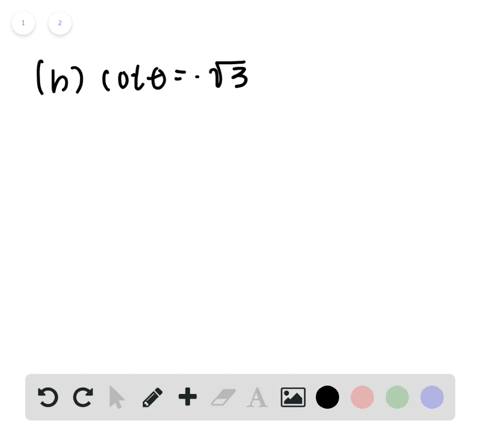🎉 The Study-to-Win Winning Ticket number has been announced! Go to your Tickets dashboard to see if you won! 🎉View Winning Ticket## Discussion

You must be signed in to discuss.

## Video Transcript

all right, Question 32 asks us to find two solutions for each of the following triggered a metric equations and then to give our answers in radiance in between zero and two pi. So, looking at the two triggered a metric equations you've been given. We noticed that both of them are c can't fada And what we know about c can't Data is, the sea can theta equals one over co signed photo. And so we need to find an angle measure honor Eunice Circle, where C camp data or one of her close and data equals two. And by now, I'm sure you guys are getting pretty familiar with your unit circle and the corresponding co sign and sign values for each radiant measure. And you might know that at multiples of pi, over four sign and co sign are both equal to, um, positive or negative variations of square root to over too. Okay. And so the reciprocal of which is equal to two. So right off the bat, we know at one over square to over two equals two divided by square, two equals two. So for part A, we want all the angles where cosign. Theta equals square root to over two and that occurs in quadrants. One in for where this coastline value is positive at hi over four and seven pi over four. So the solution part A is pi over four and seven pi over four. All right, those air to values now moving on to part B. We want to find locations were seeking data equals negative, too, or are one over coast time. Data equals negative, too, now because it's just a negative sign throwing into the mix. We can look at the other side of the unit circle where co sign still has that square root to over to value. But now it's negative. Which means when we put one negative square to over to and multiply through, we see that that is equal too negative, too. And so, for part me, um, our answers we're going to be it. Whose three pi over four in five hi over force. So answers for this one will be three pies over four in five over